Next: Implementation Up: Filtered Back Projection Previous: Filtered Back Projection

Reconstruction

One approach for reconstructing the image is simply to take the inverse Radon transform of the projections. This involves two steps; the image is back projected and then filtered with a two dimensional ramp filter.

The back projection operator,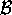is defined as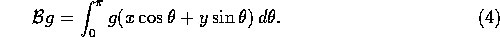This operator represents the accumulation of the projections that pass through the point (x,y). As in the example above, the back projection is a blurred version of the desired image.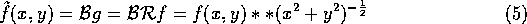The image can be now unblurred with a two-dimensional ramp filter. Because two-dimensional filtering is computationally intensive, a better a better approach is to reverse the order of the filtering and the back projection. Figure 6 illustrates both approaches. The second approach is possible due to the projection slice theorem, which states that the one-dimensional Fourier transform with respect to s of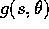is equal to the central slice at angleof the two-dimensional Fourier transform of f(x,y),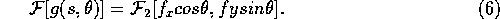The projection slice theorem implies that the Radon transform of the two-dimensional convolution of two functions is equal to the one-dimensional convolution of their Radon transforms. Convolution has the nice property of being symmetric, i.e f*g=g*f. This can be used to find a closed formula for the inverse Radon transform. It also implies a simpler inverse Radon scheme than before. It can be summarized with the following two points:

1. Do a one-dimensional high-pass filtering of each projection using a ramp filter.
2. Back project the outputs of the filter.
Note that with this approach we only need to do a one-dimensional filtering of the projections.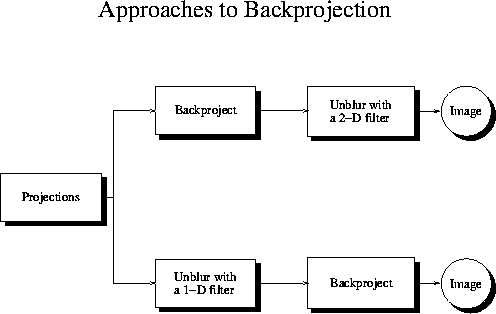Figure 6: Illustration of the two possible cases for filtered back projection. Most algorithms today does a one-dimensional filtering of the projections before the back projection.

Anders Johan Nygren
Thu May 8 12:28:25 CDT 1997